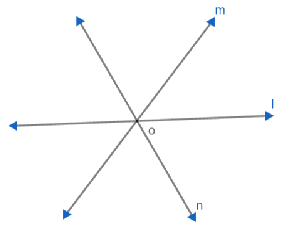When two or more lines pass through a ____ they are concurrent with each other and they are called concurrent lines.

# When two or more lines pass through a ____ they are concurrent with each other and they are called concurrent lines.

Fill Out the Form for Expert Academic Guidance!l

+91

Live ClassesBooksTest SeriesSelf Learning

Verify OTP Code (required)

### Solution:

When two or more lines pass through a single point, they are concurrent to each other and they are called concurrent lines. The point at which all the lines meet is called the point of concurrency. Let's look at the image below:Here all the lines l, m and n are intersections of O. So all these lines are parallel to each other and are therefore parallel. The point o is called the point of concurrency.

## Related content

 Area of Square Area of Isosceles Triangle Pythagoras Theorem Triangle Formula Perimeter of Triangle Formula Area Formulae Volume of Cone Formula Matrices and Determinants_mathematics Critical Points Solved Examples Type of relations_mathematics+91

Live ClassesBooksTest SeriesSelf Learning

Verify OTP Code (required)